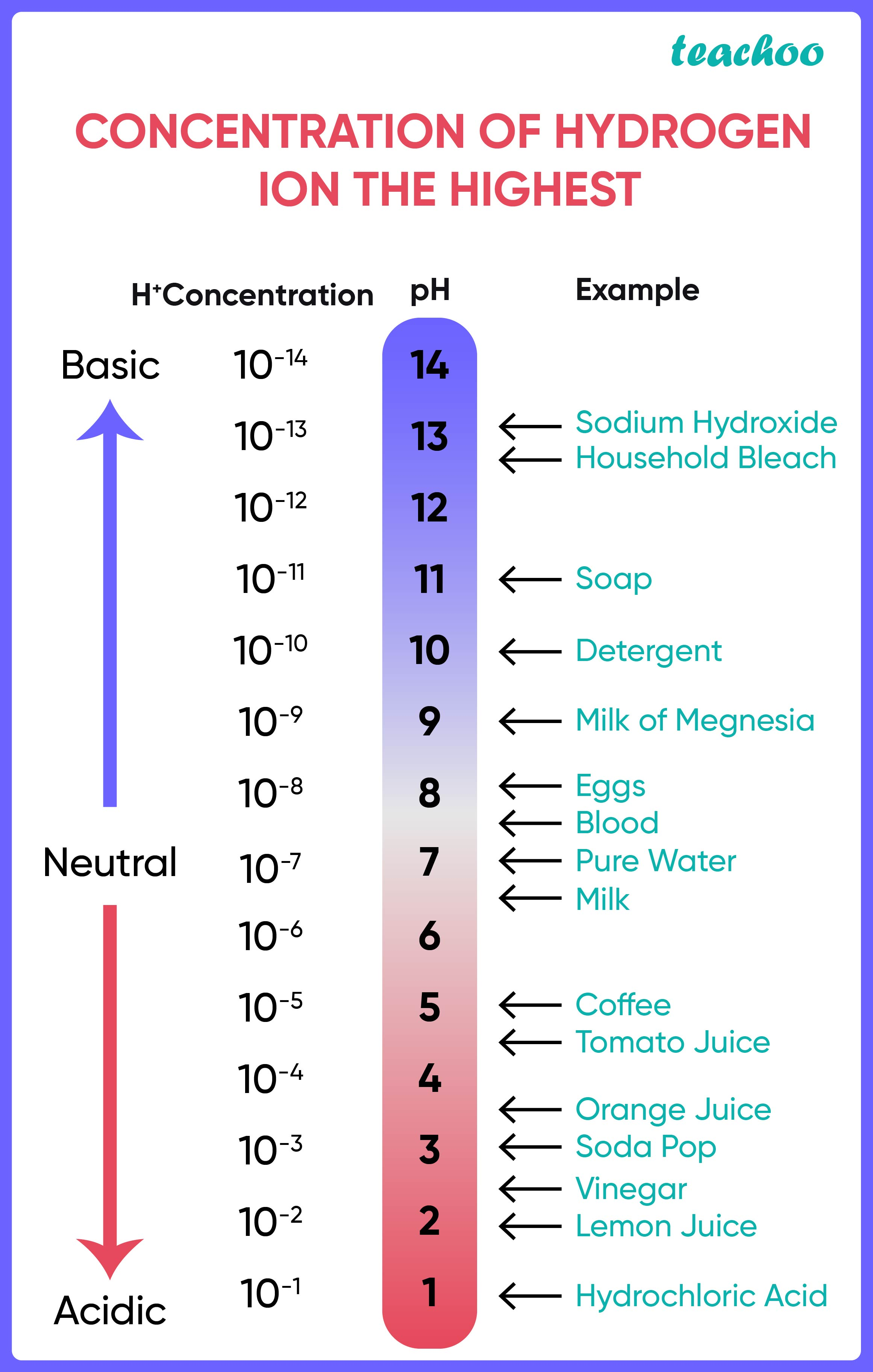Questions from inside the book

Class 10
Chapter 2 Class 10 - Acids, Bases and Salts

## You have two solutions, A and B. The pH of solution A is 6 and pH of solution B is 8. Which solution has more hydrogen ion concentration? Which of this is acidic and which one is basic?

We know that substances having pH less than 7 are acidic, and substances having pH more than 7 are basic.Therefore, A is acidic (since its pH is 6) and B is basic (Since its pH is 8) .

An acidic solution has more number of H + ions, since acids dissociate to produce H + ions.

Hence, A will have more concentration of H + ions.Introducing your new favourite teacher - Teachoo Black, at only ₹83 per month